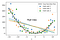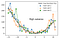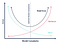# Decomposition of Bias and Variance

Once you start learning about modeling and predicting in data science, you will definitely be introduced to the idea of bias and variance and loss functions. If you’re familiar with it you know that the general idea is that high variance is a sign of overfitting and high bias is a sign of underfitting. Loss functions generally determine the loss based on the bias and variance of your model. We’re going to look at some of the math and stats behind bias and variance and how they apply to a couple of loss functions.

## Overfit and Underfit

If your model is overfit, it means that your model was trained to be so accurate for your training data that it is not properly seeing the general/overarching pattern and will not be very accurate when you predict on your testing set(or future data). In other words, it accounts for or is so tailored to the variance in the training set that you use that it will give poor predictions for any other set of data. If your model is underfit, it means that the model accounts for so little of the variance in the data, that it will be a poor predictor for both the training data and the testing or future data.

## Expectation(Statistics)

Before we dive into how bias and variance are calculated and how that factors into the loss functions for squared loss and 0–1 loss, let’s quickly review what expectation means in terms of probability and statistics.

In simple terms, the expectation is weighted average of a set of outcomes. Each outcome is weighted(multiplied) by its probability and they are all summed together. If the probability of each outcome is uniform or the same, the expectation is just the simple average or mean.

## Bias and Variance Formulas for Linear models

Bias=E[θ’]−θ or Bias=E[y’]−y (y represents the true values, y’=predicted)

Bias is calculated by taking the difference between the expectation(across training sets) of your predicted values and the actual value.Source: http://rasbt.github.io/mlxtend/user_guide/evaluate/bias_variance_decomp/

As you can see in the example above, aside from a few instances, the expectation(or average) of the predicted values is very off from the actual values so the difference along with the bias will be large.

Var(θ’)=E[θ’²]−(E[θ’])² = E[(E[θ’]−θ’)²] OR

Var(y’)=E[y’²]−(E[y’])² = E[(E[y’]−y’)²]

For calculating variance, you take the difference between the expectation of the squared predicted values and the square of the expectation of the predicted values. As you can see this is also the same as comparing the expectation of the predicted values with the predicted values.Source: http://rasbt.github.io/mlxtend/user_guide/evaluate/bias_variance_decomp/

As can be seen above, the models match up exactly within each training set, but when compared to the expectation across training sets, each prediction would have a large difference leading to a large variance and overfitting. This also shows that you can only determine bias and variance when you have predictions for multiple training sets or training and test sets, not just with one set of datapoints.

## Square Loss function

The formula for squared loss, S, is S=(y−y’)². To see how bias and variance play a part, we add and subtract E[y’] and then expand it out:

S= (y−E[y’]+E[y’]−y’)² = (y−E[y’])²+(E[y’]−y)²+2(y−E[y’])(E[y’]−y’)

Then take the expectation of both sides and get:

E[S] = E[(y−y’)²] = (y−E[y’])² + E[(E[y’]−y’)²]

As we can see, once simplified, we are left with E[S]=(Bias)² + Variance. The 2(y−E[y’])(E[y’]−y’) goes to 0 when simplified, the math can be seen here. The relationship between bias and variance can be seen more visually below. As one increases, the other decreases and the optimal model is where they’re balanced. The more to the left(lower variance/higher bias), the more underfit your model is, and the more to the right(higher variance/lower bias), the more overfit your model is.Bias-Variance tradeoff

## 0–1 Classifier Loss function

When using classification models, the loss function is a bit different and a little harder to get at first glance. First, there are some differences in how expectation, bias and variance are calculated since your predictions are only 0 or 1.

The expectation is going to be the mode instead of the mean. So you will look at the predicted classifications for a data point across training sets and whichever(0 or 1) was predicted most will be your expected classification.

The bias will still compare the expected classification to the true classification, but it will be just be 0 or 1 depending on if it matches or not. So Bias =1 if y≠E[y’], 0 otherwise.

Lastly, for variance, we are still comparing each predicted classification to the expected classification, but the variance will be the probability that the predicted classification does not match the expected one. So Variance=P(y’≠E[y’]).

For the actual loss function, we look at it in 2 cases: Bias=0 and Bias=1.

When Bias=0, the loss function is L=P(y’≠y)=0+Variance=P(y’≠E[y’]). This makes sense since if the bias is 0, the Variance should be large and should indicate overfitting.

When Bias=1, L=P(y’≠y) can be rewritten as 1−P(y’=y). Let’s take a second to understand what is happening here. When Bias=1, it means that the true classification did not match the expected(or majority) classification. However, if there are cases where y’=y, or predicted classifications match the true classification, then there are cases where y’=E[y’], or predicted classifications match the expected classification. So the cases where y’≠y are the cases where y’=E[y’]. The P(y’=E[y’])= 1−P(y’≠E[y’]) and you can rewrite L=P(y’≠y) as L=1−P(y’≠E[y’]) or 1-Variance. Bias=1 so this is also Bias-Variance.

To summarize the previous two paragraphs, when Bias=0, L=Variance=P(y’≠E[y’]) and when Bias=1,

L=Bias-Variance=1−P(y’≠E[y’]). After you think about it, this makes sense because when you have bias, it means that the expected classification does not always match the true classification so you have to subtract the variance to see how much bias there is. When there is no bias, the expected classification always matches the true classification which indicates a high variance.

For more information, take a look at the source below.

## Sources:

http://rasbt.github.io/mlxtend/user_guide/evaluate/bias_variance_decomp

Data Science student at Flatiron School

## More from Zawar Ahmed

Data Science student at Flatiron School